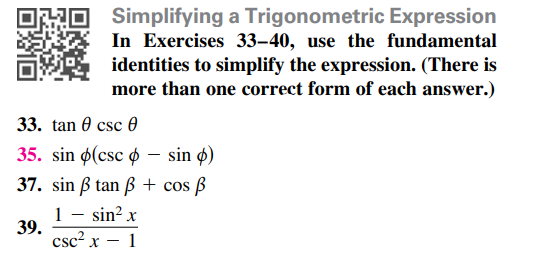### Still have math questions?

Trigonometry
QuestionSimplifying a Trigonometric Expression In Exercises $$33 - 40$$ , use the fundamental identities to simplify the expression. (There is more than one correct form of each answer.) 33. $$\tan \theta \csc \theta$$

35. $$\sin \phi ( \csc \phi - \sin \phi )$$

37. $$\sin \beta \tan \beta + \cos \beta$$

39. $$\frac { 1 - \sin ^ { 2 } x } { \csc ^ { 2 } x - 1 }$$

Simplify $$\sin ( x ) ( \csc ( x ) - \sin ( x ) ) : \cos ^ { 2 } ( x )$$

Steps

$$\sin ( x ) ( \csc ( x ) - \sin ( x ) )$$

Express with sin, cos

$$= 1 - \sin ^ { 2 } ( x )$$

Rewrite using trig identities

$$= \cos ^ { 2 } ( x )$$

Solution
View full explanation on CameraMath App.